# Maharashtra Board Class 12 Chemistry Solutions Chapter 1 Solid State

Balbharti Maharashtra State Board 12th Chemistry Textbook Solutions Chapter 1 Solid State Textbook Exercise Questions and Answers.

## Maharashtra State Board 12th Chemistry Solutions Chapter 1 Solid State

1. Choose the most correct answer.

Question i.
Molecular solids are
(a) crystalline solids
(b) amorphous solids
(c) ionic solids
(d) metallic solids
(b) amorphous solids

Question ii.
Which of the following is n-type semiconductor?
(a) Pure Si
(b) Si doped with As
(c) Si doped with Ga
(d) Ge doped with In
(b) Si doped with As

Question iii.
In Frenkel defect
(a) electrical neutrality of the substance is changed.
(b) density of the substance is changed.
(c) both cation and anion are missing
(d) overall electrical neutrality is preserved
(d) overall electrical neutrality is preserved

Question iv.
In crystal lattice formed by bcc unit cell the void volume is
(a) 68%
(b) 74%
(c) 32%
(d) 26%
(c) 32%Question v.
The coordination number of atoms in bcc crystal lattice is
(a) 2
(b) 4
(c) 6
(d) 8
(d) 8

Question vi.
Which of the following is not correct ?
(a) Four spheres are involved in the formation of tetrahedral void.
(b) The centres of spheres in octahedral voids are at the a pices of a regular tetrahedron.
(c) If the number of atoms is N the number of octahedral voids is 2N.
(d) If the number of atoms is N/2, the number of tetrahedral voids is N.
(c) If the number of atoms is N the number of octahedral voids is 2N.

Question vii.
A compound forms hcp structure. Number of octahedral and tetrahedral voids in 0.5 mole of substance is respectively
(a) 3.011 × 1023, 6.022 × 1023
(b) 6.022 × 1023, 3.011 × 1023
(c) 4.011 × 1023, 2.011 × 1023
(d) 6.011 × 1023, 12.022 × 1023
(a) 3.011 × 1023, 6.022 × 1023

Question viii.
Pb has fcc structure with edge length of unit cell 495 pm. Radius of Pb atom is
(a) 205 pm
(b) 185 pm
(c) 260 pm
(d) 175 pm
(d) 175 pm2. Answer the following in one or two sentences.

Question i.
What are the types of particles in each of the four main classes of crystalline solids?
The smallest constituents or particles of various solids are atoms, ions or molecules.

Question ii.
Which of the three types of packing used by metals makes the most efficient use of space and which makes the least efficient use ?
fcc has the most efficient packing of particles while scc has the least efficient packing.

Question iii.
The following pictures show population of bands for materials having different electrical properties. Classify them as insulator, semiconductor or a metal.Picture A represents metal conductor,
Picture B represents insulator,
Picture C represents semiconductor.

Question iv.
What is a unit cell?

• Unit cell : It is the smallest repeating structural unit of a crystalline solid (or crystal lattice) which when repeated in different directions produces the crystalline solid (lattice).
• The crystal is considered to consist of an infinite number of unit cells.
• The unit cell possesses all the characteristics of the crystalline solid.Question v.
How does electrical conductivity of a semiconductor change with temperature ? Why?

• Since the energy difference between valence band and conduction band in semiconductor is not large, the electrons from valence band can be promoted to conduction by heating.
• Hence electrical conductivity of a semiconductor increases with temperature.

Question vi.
The picture represents bands of MOs for Si. Label valence band, conduction band and band gap.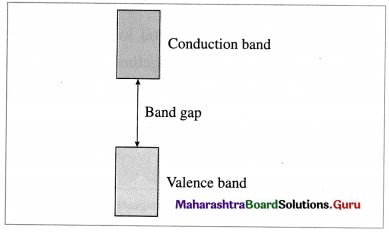Question vii.
A solid is hard, brittle and electrically non-conductor. Its melt conducts electricity. What type of solid is it?
A solid crystalline electrolyte like NaCl is hard, brittle and electrically nonconductor. But its melt conducts electricity.

Question viii.
Mention two properties that are common to both hep and ccp lattices.
In hcp and ccp crystal lattices coordination number is 12 and packing efficiency is 74%.

Question ix.
Sketch a tetrahedral void.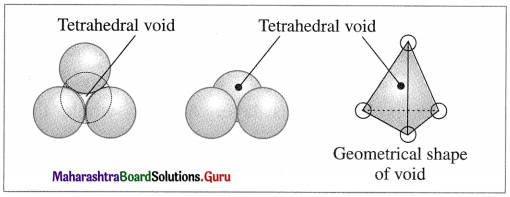Question x.
What are ferromagnetic substances?

1. The substances which possess unpaired electrons and high paramagnetic character and when placed in a magnetic field are strongly attracted and show permanent magnetic moment even when the external magnetic field is removed are said to be ferromagnetic. They can be permanently magnetised.
2. In the solid state, the metal ions of ferromagnetic substance are grouped together into small regions called domains, where each domain acts as a tiny magnet.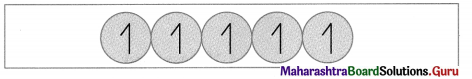For example : Fe, Co, Gd, Ni, CrO2, etc.

3. Answer the following in brief.

Question i.
What are valence band and conduction band?
There are two types of bands of molecular orbitals as follows :

• Valence band : The atomic orbitals with filled electrons from the inner shells form valence bands, where there are no free mobile electrons since they are involved in bonding.
• Conduction band : Atomic orbitals which are partially filled or empty on overlapping form closely placed molecular orbitals giving conduction bands where electrons are delocalised and can conduct, heat and electricity.

Question ii.
Distinguish between ionic solids and molecular solids.

 Type/ Property Ionic solids Molecular solids 1. Particles of unit cell Cations and anions Monoatomic or polyatomic molecules 2. Interparticle forces Electrostatic London, dipole-dipole forces and/or hydrogen bonds 3. Hardness Hard and brittle Soft 4. Melting points High 600 °C to 3000 °C Low (-272 °C to 400 °C) 5. Thermal and electrical conductivity Poor electrical conductors in solid state. Good conductors when melted or dissolved in water. Poor conductor of heat and electricity 6. Examples NaCl, CaF2 ice, benzoic acid

Question iii.
Calculate the number of atoms in fcc unit cell.
Number of atoms in face-centred cubic (fcc) unit cell :
In this unit cell, there are 8 atoms at 8 corners and 6 atoms at 6 face centres. Each corner contributes 1/8th atom to the unit cell, hence due to 8 corners,
Number of atoms = $$\frac {1}{8}$$ × 8 = 1.
Each face centre contributes half of the atom to the unit cell, hence due to 6 face centres,
Number of atoms = $$\frac {1}{2}$$ × 6 = 3.
∴ Total number of atoms present in fee unit cell = 1 + 3 = 4.
Hence the volume of the unit cell is equal to the volume of four atoms.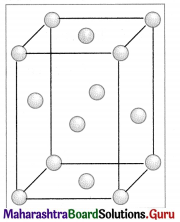Face centered unit cell

Question iv.
How are the spheres arranged in first layer of simple cubic close-packed structures? How are the successive layers of spheres placed above this layer ?
(i) Stacking of square close packed layers :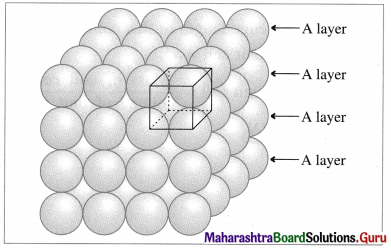Stacking of square close packed layers

In this arrangement, the two dimensional AAAA type square closed packed layers are placed one over the other in such a way that the crests of all spheres are in contact with successive layers in all directions. All spheres of different layers are perfectly aligned horizontally and vertically forming unit cells having primitive or simple cubic structure. Since all the layers are identical and if each layer is labelled as layer A, then whole three dimensional crystal lattice will be of AAAA… type.

Each sphere is in contact with six surrounded spheres, hence the coordination number of each sphere is six.

(ii) Stacking of two hexagonal close packed layers :
A close packed three dimensional structure can be generated by arranging hexagonal close packed layers in a particular manner.

In this the spheres of second layer are placed in the depression of the first layer.
In this if first layer is labelled as A then second layer is labelled as B since they are aligned differently.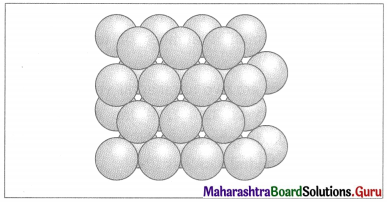Two layers of closed packed spheres

In this, all triangular voids of the first layers are not covered by the spheres of the second layer. The triangular voids which are covered by second layer spheres generate tetrahedral void which is surrounded by four spheres. The triangular voids in one layer have above them triangular voids of successive layers.

The overlapping triangular voids from two layers together form an octahedral void which is surrounded by six spheres.Question v.
Calculate the packing efficiency of metal crystal that has simple cubic structure.
Step 1 : Radius of sphere : In simple cubic lattice, the atoms (spheres) are present at eight corners and in contact along the edge in the unit cell.
If ‘a’ is the edge length of the unit cell and ‘r’ is the radius of the atom, then
a = 2r or r = a/2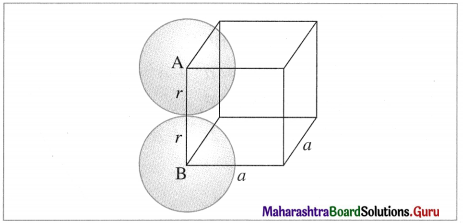scc structure

Step 2 : Volume of sphere :
Volume of one particle = $$\frac{4 \pi}{3}$$ × r3
= $$\frac{4 \pi}{3}$$ × (a/2)3 = $$\frac{\pi a^{3}}{6}$$

Step 3 : Total volume of particles : Since the unit cell contains one particle. Volume occupied by one particle in unit cell = $$\frac{\pi a^{3}}{6}$$

Step 4 : Packing efficiency :
Packing efficiency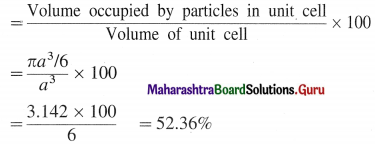∴ Packing efficiency = 52.36%
Percentage of void space = 100 – 52.36
= 47.64%

Question vi.
What are paramagnetic substances? Give examples.
(1) The magnetic properties of a substance arise due to the presence of electrons.
(2) An electron while revolving around the nucleus, also spins around its own axis and generates a magnetic moment and magnetic properties.
(3) If an atom or a molecule contains one or more unpaired electrons spinning in same direction, clockwise or anticlockwise, then the substance is associated with net magnetic moment and magnetic properties. They experience a net force of attraction when placed in the magnetic field. This phenomenon is called paramagnetism and the substance is said to be paramagnetic.
For example, O2, Cu2+, Fe3+ , Cr3+ , NO, etc.

Question vii.
What are the consequences of Schottky defect?
Consequences of Schottky defect :

• Since the number of ions (cations and anions) decreases but volume remains unchanged, the density of a substance decreases.
• As the number of missing cations and anions is equal, the electrical neutrality of the compound remains same.
• This defect arises in ionic crystals like NaCl, AgBr, KCl, etc.

Question viii.
Cesium chloride crystallizes in cubic unit cell with Cl ions at the corners and Cs+ ion in the centre of the cube. How many CsCl molecules are there in the unit cell ?
Number of Cs+ ion at body centre = 1
Number of Cl ions due to 8 comers = 8 × $$\frac {1}{8}$$ = 1
Hence unit cell contains 1 CsCl molecule.Question ix.
Cu crystallizes in fee unit cell with edge length of 495 pm. What is the radius of Cu atom ?
Given : a = 495 pm
For fee structure,
radius = r = $$\frac{a}{2 \sqrt{2}}=\frac{495}{2 \times \sqrt{2}}$$ = 175 cm.
Radius of Cu atom = 175 pm

Question x.
Obtain the relationship between density of a substance and the edge length of unit cell.
(1) Consider a cubic unit cell of edge length ‘a’.
The volume of unit cell = a3

(2) If there are ‘n’ particles per unit cell and the mass of particle is ‘m’, then
Mass of unit cell = m × n.

(3) If the density of the unit cell of the substance is p then
Density of unit cell = $$\frac{\text { Mass of unit cell }}{\text { Volume of unit cell }}$$
ρ = $$\frac{m \times n}{a^{3}}$$

Question 4.
The density of iridium is 22.4 g/cm3. The unit cell of iridium is fcc. Calculate the radius of iridium atom. Molar mass of iridium is 192.2 g/mol.
Given : Crystal structure of iridium = fcc
Molar mass of iridium = 192.2 gmol-1
Density = ρ = 22.4 gcm-3
In fcc structure, there are 8 Ir atoms at 8 comers and 6 Ir atoms at 6 face centres.
∴ Total number of Ir atoms = $$\frac {1}{8}$$ × 8 + $$\frac {1}{2}$$ × 6
= 1 + 3
= 4
Mass of Ir atom = $$\frac{192.2}{6.022 \times 10^{23}}$$
= 31.92 × 10-23 g
∴ Mass of 4 Ir atoms = 4 × 31.92 × 10-23
= 1.277 × 10-21 g
∴ Mass of unit cell = 1.277 × 10-21 g
Density of unit cell = $$\frac{\text { Mass of unit cell }}{\text { Volume of unit cell }}$$
22.4 = $$\frac{1.277 \times 10^{-21}}{a^{3}}$$
∴ a3 = $$\frac{1.277 \times 10^{-21}}{22.4}$$
= 57 × 10-24 cm3
∴ a = (57 × 10-24)3 = 3.848 × 10-8 cm
If r is the radius of iridium atom, then for fcc structure,
r = $$\frac{a}{2 \sqrt{2}}$$
= $$\frac{3.848 \times 10^{-8}}{2 \times 1.414}$$
= 1.36 × 10-8 cm
= 136 pm
Radius of iridium atom = 136 pm

Question 5.
Aluminium crystallizes in cubic close packed structure with unit cell edge length of 353.6 pm. What is the radius of Al atom ? How many unit cells are there in 1.00 cm3 of Al ?
Given : Structure of Al
= Cubic close packed structure
= ccp structure
Edge length of unit cell = a = 353.6 pm
= 3.536 × 10-8 cm
r = ?
Number of unit cells in 1.00 cm3 of Al = ?
Radius of Al atom = r = $$\frac{a}{2 \sqrt{2}}=\frac{353.6}{2 \sqrt{2}}$$
= $$\frac{353.6}{2 \times 1.414}$$ = 125 pm
Volume of one unit cell = a3 = (3.536 × 10-8)3
= 4.421 × 10-23 cm3
Number of unit cells = $$\frac{1.00}{4.421 \times 10^{-23}}$$
= 2.26 × 1022
Radius of Al atom = 125 pm
Number of unit cells = 2.26 × 1022Question 6.
In an ionic crystalline solid atoms of element Y form hcp lattice. The atoms of element X occupy one third of tetrahedral voids. What is the formula of the compound?
In the given hcp lattice, Y atoms are present at 12 corners and 2 face centres.
∴ Number of Y atoms = $$\frac {1}{2}$$ × 12 + 2 × $$\frac {1}{2}$$ = 3
There are 6 tetrahedral voids, the number of X atoms = $$\frac {1}{3}$$ × 6 = 2
∴ Formula of the compound is X2Y3.

Question 7.
How are tetrahedral and octahedral voids formed?
Tetrahedral void : The vacant space or void among four constituent particles having tetrahedral arrangement in the crystal lattice is called tetrahedral void.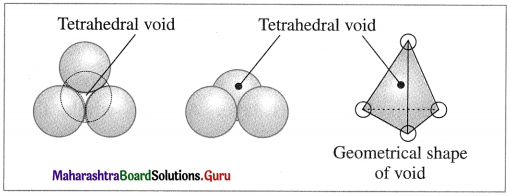The arrangement of four spheres around the void is tetrahedral. A tetrahedral void is formed when a triangular void made by three coplanar spheres is in contact with fourth sphere above or below it.

Octahedral void : The vacant space or void at the centre of six spheres (or atoms) which are placed octahedrally is called octahedral void.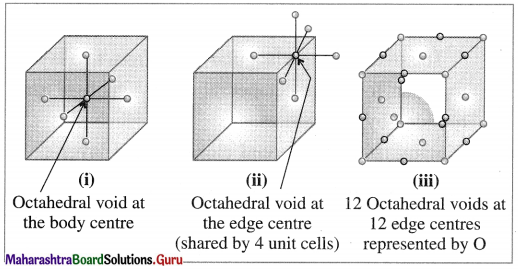Question 8.
Third layer of spheres is added to second layer so as to form hcp or ccp structure. What is the difference between the addition of third layer to form these hexagonal close-packed structures?

1. In the formation of hexagonal closed-packed (hcp) structure, the first one dimensional row shows depressions between neighbouring atoms.
2. When a second row is arranged so that spheres fit in these depressions then a staggered arrangement is obtained. If the first row is A then the second row is B.
3. When third row is placed in staggered manner in contact with second row then A type arrangement is obtained.
4. Similarly, the spheres in fourth row can be arranged as B type layer. This results in ABAB… type setting of the layers. This gives hexagonal close packing (hcp) structure.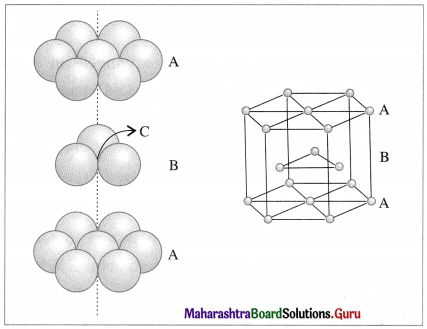Hexagonal close packing (hcp)

Question 9.
An element with molar mass 27 g/mol forms cubic unit cell with edge length of 405 pm. If density of the element is 2.7 g/cm3, what is the nature of cubic unit cell ? (fcc or ccp)
Given : Molar mass = M = 27 g mol-1
Nature of crystal = cubic unit cell
Edge length = a = 405 pm = 4.05 × 10-8 cm
Density = ρ = 2.7 g cm-3
Nature of unit cell = ?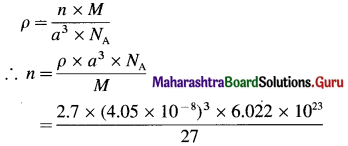= 3.997
≅ 4
Hence the nature of unit cell = face-centred cubic unit cell
Radius of Al atom = 125 pm
The nature of cubic unit cell is fcc.Question 10.
An element has a bcc structure with unit cell edge length of 288 pm. How many unit cells and number of atoms are present in 200 g of the element? (1.16 × 1024, 2.32 × 1024)

Question 11.
Distinguish with the help of diagrams metal conductors, insulators and semiconductors from each other.
Conductor:

1. A substance which conducts heat and electricity to a greater extent is called conductor.
2. In this, conduction bands and valence bands overlap or are very closely spaced.
3. There is no energy difference or very less energy difference between valence bands and conduction bands.
4. There are free electrons in the conduction bands.
5. The conductance decreases with the increase in temperature.
6. E.g., Metals, alloys.
7. The conducting properties can’t be improved by adding third substance.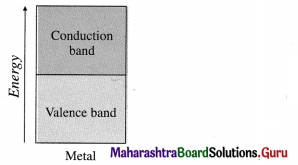Insulator:

1. A substance which cannot conduct heat and electricity under any conditions is called insulator.
2. In this, conduction bands and valence bands are far apart.
3. The energy difference between conduction bands and valence bands is very large.
4. There are no free electrons in the conduction bands and electrons can’t be excited from valence bands to conduction bands due to large energy difference.
5. No effect of temperature on conducting properties.
6. E.g., Wood, rubber, plastics.
7. No effect of addition of any substance.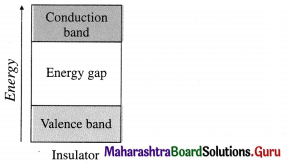Semiconductor:

1. A substance that has poor electrical conductance at low temperature but higher conductance at higher temperature is called semiconductor.
2. In this, conduction bands and valence bands are spaced closely.
3. The energy difference between conduction bands and valence bands is small.
4. The electrons can be easily excited from valence bands to conduction bands by heating.
5. Conductance increases with the increase in temperature.
6. E.g., Si, Ge
7. By doping, conducting properties improve. E.g. n-type, p-type semiconductors.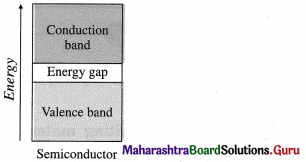Question 12.
What are n-type semiconductors? Why is the conductivity of doped n-type semiconductor higher than that of pure semiconductor ? Explain with diagram.
n-type semiconductor:

• n-type semiconductor contains increased number of electrons in the conduction band.
• When Si semiconductor is doped with 15th group element phosphorus, P, the new atoms occupy some vacant sites in the lattice in place of Si atoms.
• P has five valence electrons, out of which four are involved in covalent bonding with neighboring Si atoms while one electrons remains free and delocalised.
• These free electrons increase the electrical conductivity of the semiconductor.
• The semiconductors with extra non-bonding free electrons are called n-type semiconductors.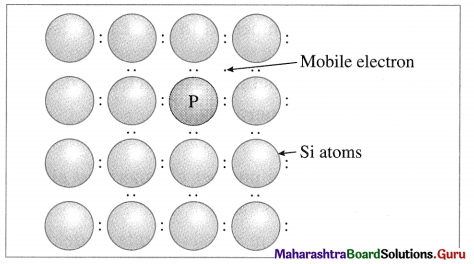P atom occupying regular site of Si atom

Question 13.
Explain with diagram. Frenkel defect. What are the conditions for its formation? What is its effect on density and electrical neutrality of the crystal?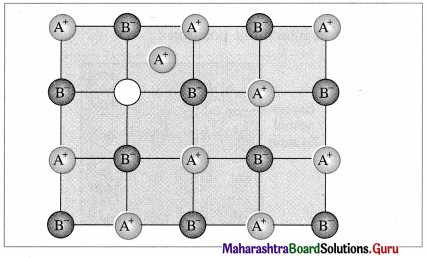1. Frenkel defect : This defect arises when an ion of an ionic compound is missing from its regular site and occupies interstitial vacant position between lattice points.
2. Cations have smaller size than anions, hence generally cations occupy the interstitial sites.
3. This creates a vacancy defect at its original position and interstitial defect at new position.
4. Frenkel defect is regarded as the combination of interstitial defect and vacancy defect.

Conditions for the formation of Frenkel defect :

1. This defect arises in ionic compounds with a large difference between the sizes of cation and anion.
2. The ionic compounds must have ions with low coordination number.

Consequences of Frenkel defect :

1. Since there is no loss of ions from the crystal lattice, the density of the solid remains unchanged.
2. The crystal remains electrically neutral.
3. This defect is observed in ZnS, AgCl, AgBr, Agl, CaF2, etc.Question 14.
What is an impurity defect? What are its types? Explain the formation of vacancies through aliovalent impurity with example.
Impurity defect : This defect arises when foreign atoms, that is, atoms different from the host atoms are present in the crystal lattice.

There are two types of impurity defects namely

1. Substitutional defects and
2. Interstitial defects.

(1) Substitutional defects : These defects arises when foreign atoms occupy the lattice sites in place of host atoms, due to their displacements.
Examples : Solid solutions of metals (alloys). For example. Brass in which host atoms are of Cu which are replaced by impurity of Zn atoms. In this Zn atoms occupy regular sites while Cu atoms occupy substituted sites.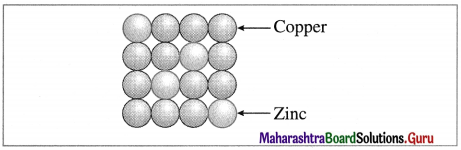Brass

Vacancy through aliovalent impurity :
By addition of impurities of aliovalent ions :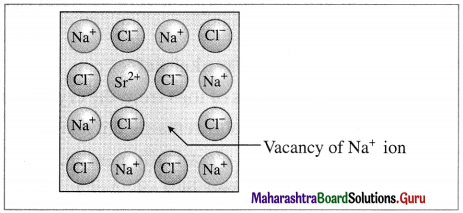Vacancy through aliovalent ion

When aliovalent ion like Sr2+ in small amount is added by additing SrCl2 to NaCl during its crystallisation, each Sr2+ ion (oxidation state 2+) removes 2 Na+ ions from their lattice points, to maintain electrical neutrality. Hence one of vacant lattice site is occupied by Sr2+ ion while other site remains vacant.

Interstitial impurity defect :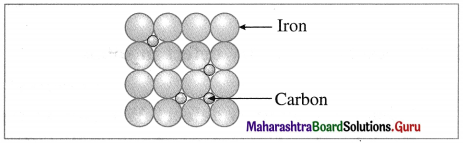Stainless steel

A defect in solid in which the impurity atoms occupy interstitial vacant spaces of lattice structure is called interstitial impurity defect.

For example, in steel, normal lattice sites are occupied by Fe atoms but interstitial spaces are occupied by carbon atoms.12th Chemistry Digest Chapter 1 Solid State Intext Questions and Answers

Try this… (Textbook Page No. 1)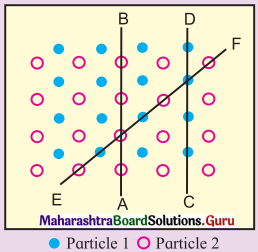Observe the above figure carefully. The two types of circles in this figure represent two types of constituent particles of a solid.

Question 1.
Will you call the arrangement of particles in this solid regular or irregular ?
The arrangement of particles in this solid is regular.

Question 2.
Is the arrangement of constituent particles in directions $$\overrightarrow{\mathbf{A B}}$$, $$\overrightarrow{\mathbf{C D}}$$ and $$\overrightarrow{\mathbf{E F}}$$ same or different?
$$\overrightarrow{\mathbf{A B}}$$ represents arrangement of identical particles of one type.
$$\overrightarrow{\mathbf{C D}}$$ represents arrangement of identical particles of another type.
$$\overrightarrow{\mathbf{E F}}$$ represents regular arrangement of two different particles in alternate positions.

Use your brain power ! (Textbook Page No. 2)

Question 1.
Identify the arrangements A and B as crystalline or amorphous.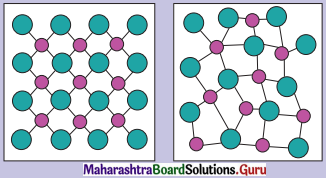Arrangement in image A indicates the substance is crystalline.
Arrangement in image B indicates the substance is amorphous.

Try this… (Textbook Page No. 3)

Question 1.
Graphite is a covalent solid yet soft and good conductor of electricity. Explain.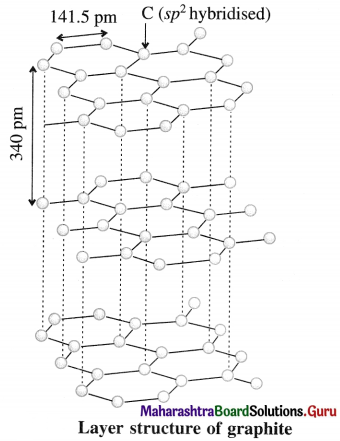1. Each carbon atom in graphite is sp2 hybridised and covalently bonded to other three sp2 hybridised carbon atoms forming σ bonds and the fourth electron in 2pz orbital of each carbon atom is used in the formation of a π bond. This results in the formation of hexagonal rings in two dimensions.
2. In graphite, the layers consisting of hexagonal carbon network are held together by weak van der Waal’s forces imparting softness.
3. The electrons in π bonds in the ring are delocalised and free to move in the delocalised molecular orbitals giving good electrical conductance.

Use your brain power ! (Textbook Page No. 13)

Question 1.
Which of the three lattices scc, bcc and fcc has the most efficient packing of particles ? Which one has the least efficient packing ?
fcc has the most efficient packing of particles while see has the least efficient packing.

Can you think ? (Textbook Page No. 20)

Question 1.
When ZnO is heated it turns yellow and returns back to original white colour on cooling. What could be the reason ?
When colourless ZnO is strongly heated, the metal atoms are deposited on crystal surface and anions O2- migrate to the surface producing vacancies at anion lattice points.

These anions combine with Zn atoms forming ZnO and release electrons.
Zn + O2- → ZnO + 2e

These released electrons diffuse into the crystal and occupy vacant sites of anions and produce F- centres. Due to these colour centres, ZnO turns yellow.

Can you tell ? (Textbook Page No. 23)

Let a small quantity of phosphorus be doped into pure silicon.

Question 1.
Will the resulting material contain the same number of total number of electrons as the original pure silicon ?